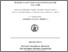Ricerca
Browse
Informazioni

Del Prete, Ida (2006) Efficient numerical methods for Volterra integral equations of Hammerstein type. [Tesi di dottorato] (Unpublished)Preview
PDF
Del_Prete_Dottorato.pdf

[error in script] [error in script]
Item Type: Tesi di dottorato
Lingua: English
Title: Efficient numerical methods for Volterra integral equations of Hammerstein type
Creators:
CreatorsEmail
Del Prete, IdaUNSPECIFIED
Date: 2006
Date Type: Publication
Number of Pages: 166
Institution: Università degli Studi di Napoli Federico II
Department: Matematica e applicazioni "Renato Caccioppoli"
Dottorato: Scienze computazionali e informatiche
Ciclo di dottorato: 18
Coordinatore del Corso di dottorato:
nomeemail
Ricciardi, Luigi MariaUNSPECIFIED
Tutor:
nomeemail
Russo, ElviraUNSPECIFIED
Date: 2006
Number of Pages: 166
Uncontrolled Keywords: Volterra integral equations, fast numerical methods, discrete Volterra equations
Settori scientifico-disciplinari del MIUR: Area 01 - Scienze matematiche e informatiche > MAT/08 - Analisi numerica
Date Deposited: 30 Jul 2008
URI: http://www.fedoa.unina.it/id/eprint/731
DOI: 10.6092/UNINA/FEDOA/731## Abstract

Volterra integral equations (VIEs) are the mathematical model of many evolutionary problems with memory arising from biology, chemistry, physics, engineering. It is known that the numerical treatment of VIEs has an high computational cost, due mainly to the computation of the ``lag term'' or ``tail term'' which contains the past history of the phenomenon. Since it depends on time, the ``lag term'' has to be computed for each time step and its cost increases when time passes. Among the Volterra equations, the Hammerstein type ones, are particularly interesting for the applications. The aim of this thesis is the construction of numerical methods for VIEs of Hammerstein type which produce accurate solution at a low computational cost and ``catch'' the qualitative behaviour of the exact solution. The study developed has been concerned at first with the construction and analysis of efficient methods for the numerical treatment of VIEs of Hammerstein type where the Laplace transform of the kernel rather than the convolution kernel itself is a priori known. This is not an anomalous or restricting situation, as a matter of fact these kind of problems arise in chemical absorption kinetics in the determination of non reflecting boundary conditions, and in general in situations when Laplace transform tecnique are used to reduce systems of ordinary or partial differential equations in VIEs. It is known that a classical numerical method for computing the numerical solution of such equations over Nt time steps requires O(N2t) operations and O(Nt) memory space. In this thesis we construct two classes of fast numerical methods based on collocation and Runge-Kutta formulas respectively. These methods have a computational cost of O(NtlogNt) operations, O(logNt) memory requirement and they have an high order of accuracy. In both cases the knowledge of the Laplace transform of the kernel and the convolution nature of the kernel itself are exploited in order to obtain a fast computation of the lag term. This is possible by using an opportune inverse Laplace transform approximation formula for computing the kernel evaluations. The fast numerical methods constructed in this thesis tend to the corresponding classical methods when the inverse Laplace transfrom approximation formula is exact. The convergence analysis of the fast collocation and Runge-Kutta methods shows that their order of convergence coincides with the order of the corresponding classical methods. We also analyse the stability properties of the fast Runge-Kutta methods with respect to test equations. We prove that the stability regions depend on the approxiamation of the inverse Laplace transform and that the stability properties of the classical Runge-Kutta methods are obtained when the error of the inverse Laplace transform approximation formula tends to zero. The numerical experiments on some significant problems taken from the ``Test Set'' collection project confirm the expected accuracy, computational cost and the stability properties of the constructed methods. The second part of the thesis concerns with the numerical treatment of problems of SIS epidemic diffusion with periodic immigration flow. The mathematical model of such problems is represented by an Hammerstein type VIE with convolution kernel. We consider problems caracterized by the relapse of the epidemic which implies that the VIE has an asymptotically periodic solution. It is clear that an efficient numerical method has to reproduce the asymptotically periodic solution whenever applied to equations that show this behaviour. For this reason we analyse the discrete Volterra equation (DVE) corresponding to such VIEs and we prove a theorem which establishes the existence and the uniqueness of the asymptotically periodic solution of the DVE. Moreover we consider SIS epidemic models with periodic immigration flow and constant contact rate. Also in this case we prove, for the DVE corresponding to the problem, the existence and the uniqueness of the asymptotically periodic solution when the DVE satisfies some significant hypothesis depending only on its kernel and forcing term. In order to analyse if the existing numerical methods satisfy these conditions, that is if they are AP-stable, we consider the class of θ-methods and we prove that they are AP-stable if the integration step satisfies an inequality depending only on some parameters that are characteristic of the problem.